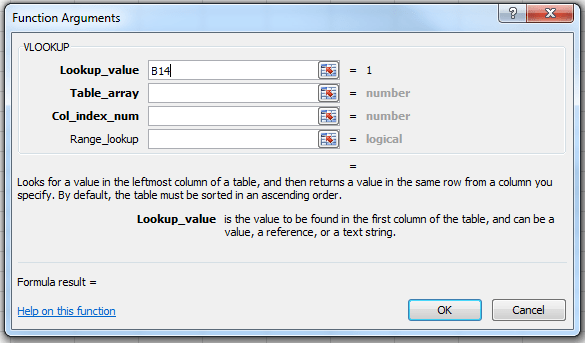# How To Use Vlookup & Hlookup Functions In Excel

VLOOKUP and HLOOKUP are functions in Excel that allow you to search a table of data and based on what the user has supplied and give appropriate information from that table.

If you have a table of Student ID numbers, Student Names and Grades, you can set up Excel so that if a Student ID number is supplied by the user, it will look through the table and output the student’s name and grade.

VLOOKUP allows you to search a table that is set up vertically. That is, all of the data is set up in columns and each column is responsible for one kind of data. In the Student Record example, there would be a separate column of data for Student Names, one for Student ID numbers, etc.

HLOOKUP is the exact same function, but looks up data that has been formatted by rows instead of columns.

The format of the VLOOKUP function is: VLOOKUP(lookup_value,table_array,col_index_num,range_lookup).

The lookup_value is the user input. This is the value that the function uses to search on. If you are searching a table by the Student ID number, then the lookup_value is the cell that contains the inputted Student ID number being looked up.

The table_array is the area of cells in which the table is located. This includes not only the column being searched on, but the data columns for which you are going to get the values that you need. Back to the example, the table_array would not only include the Student ID number column, but the columns for the Student Names and Grades as well.

The col_index_num is the column of data that contains the answer that you want. If your table is set up as: column 1 – Student ID Number, column 2 – Student Names, column 3 – Grades and you inputted a Student ID Number and you want to retrieve the grade that was received for that person, the col_index_num would be 3. 3 is the column number of the data column for the ANSWER that you are trying to look up.

Range_lookup is a TRUE or FALSE value. When set to TRUE, the lookup function gives the closest match to the lookup_value without going over the lookup_value. When set to FALSE, an exact match must be found to the lookup_value or the function will return #N/A. Note, this requires that the column containing the lookup_value be formatted in ascending order.

#### To use the Function Wizard to insert a VLOOKUP function:

1. Select the cell that will contain the answer to the VLOOKUP and access the Insert Function dialog, which depends upon the version of Excel that you are using:
• For Excel 2007 and 2010: Go to the Formula tab on the ribbon, and choose Insert Function.
• For Excel 2003 and earlier: Select Insert -> Function…
2. Under the Function Category, choose either All or Lookup & Reference.
3. Under the Function Name, select VLOOKUP, and hit OK.1. The Function Wizard for VLOOKUP will then display. The 4 values talked about above (lookup_value, table_array, col_index_num, range_lookup) are required by the function. Each line for each value required. If you put the cursor into the first line for lookup_value, down below it explains what the lookup_value is for your reference. Similar information is displayed when the cursor is in any of the other fields.1. Enter in the lookup_value either by typing in the number for the cell, or, by selecting the cell on the worksheet.
2. Enter in the table_array by typing in the numbers for the cells, or, by selecting the group of cells on the worksheet.
3. Enter in the number for column which contains the data that you wish to obtain in the col_index_num area.
4. Enter into the range_lookup field the value TRUE if the function should accept the closest value to your lookup_value without going over or FALSE if an exact match is required.